# Constructions and congruency

### Term 1 starting in week 10 :: Estimated time: 3 weeks

• Draw and measure angles (review)
• Construct and interpret scale drawings (review)
• Locus of distance from a point
• Locus of distance from a straight line/shape
• Locus equidistant from two points
• Construct a perpendicular bisector
• Construct a perpendicular from a point
• Construct a perpendicular to a point
• Locus of distance from two lines
• Construct an angle bisector
• Construct triangles from given information (review)
• Identify congruent figures
• Explore congruent triangles
• Identify congruent triangles

This page should remember your ticks from one visit to the next for a period of time. It does this by using Local Storage so the information is saved only on the computer you are working on right now.

## Lesson Starters

Here are some suggestions for whole-class, projectable resources which can be used at the beginnings of each lesson in this block.

### 1st Lesson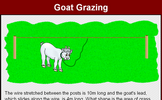#### Goat Grazing

Find the loci of the goat's position as it eats the grass while tethered to the rope.

### 2nd Lesson#### The Power of Christmas

Find a power of 2 and a power of 3 that are consecutive numbers.

### 3rd Lesson#### Christmas Eve

Is there a pattern in the number of palindromic numbers to be found less than powers of 10?

### 4th Lesson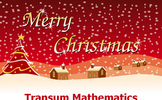#### Christmas Tables

Which of the two shapes has the largest area? You will be surprised!

### 5th Lesson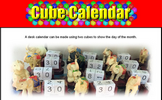#### Cube Calendar

What numbers should be on each face of the two cubes to make this perpetual calendar?

### 6th Lesson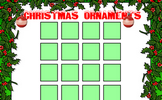#### Christmas Ornaments

A Maths Starter activity requiring students to arrange Christmas ornaments in a square box.

### 7th Lesson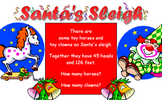#### Santa's Sleigh

Work out the number of clowns and horses given the number of heads and feet.

### 8th Lesson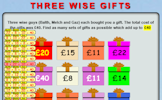Find as many sets of three of the available numbers as possible which add up to the given total.

### 9th Lesson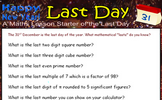#### Last Day

The 31st of December is the last day of the year. What mathematical lasts do you know?

Some of the Starters above are to reinforce concepts learnt, others are to introduce new ideas while others are on unrelated topics designed for retrieval practice or and opportunity to develop problem-solving skills.

White Rose ResourcesEnd of block assessments provide a quick progress check at the end of each block of learning to make sure students have understood the content covered. This Scheme of Learning was produced by White Rose Maths and is used here with permission granted on 30th June 2021.For All: